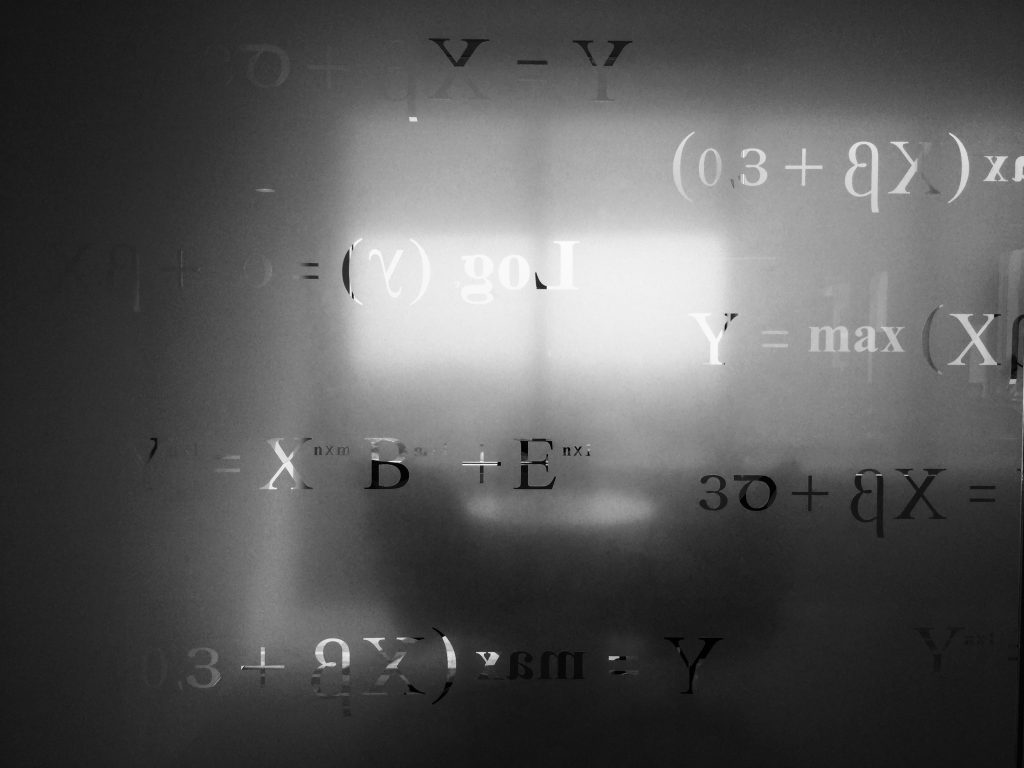# The “new” fundamental arithmetic operations

Sums, products and powers have terrified almost all elementary and middle school students in time. But how many of you knew they aren’t the only basic arithmetic operations?

During my now over career as a student, one of the attitudes that I encountered with distressing frequency is that of preconceived intolerance towards mathematics. You will certainly have heard phrases such as: “I’m not interested in these topics because they won’t be of any use to me, useful mathematics is studied in middle school”. Not infrequently, however, these beliefs are fueled by a poor teaching system that focuses on notionism and fails to arouse and keep the interest of learners awake.

Mathematics, far from being a discipline fossilized on a handful of sterile theorems, dynamically evolves to respond to the practical and abstract needs of an increasingly sophisticated world. In this article some recently formulated and neglected topics in current educational programs will be briefly illustrated, although they can be categorized as “basic”.

Tetraction

The tetraction represents the fourth fundamental arithmetic operation (after the best-known algebraic sum, product and power) and the simplest of the so-called hyper-operations. It consists, as the latter expression suggests, in a compact notation to represent a power characterized by a series of exponents rather than just one. Its most relevant application is therefore to easily represent huge numbers, as often happens in the field of information technology. The operation consists in raising the base for an exponent which is obtained in turn by raising it to itself a number of times indicated by the index at the top left:

(Image source and definitions: Wikipedia; it reads: “the nth tetration of a” or “nth root of a”)

Hyperfactorial and superfactorial

By hyperfactorial of a natural number n is meant the product of positive integers less than or equal to this number, each raised to a power equal to itself:

On the other hand, the superfactorial has multiple definitions:

1. According to mathematicians Neil Sloane and Simon Plouffe (Encyclopedia of Integer Sequences, 1995), the superfactorial of a natural number n is defined as the product of the factorial numbers of integers less than or equal to that number:

• Taking advantage of the tetraction operation mentioned above, Clifford Pickover (Keys to Infinity, 1995) proposed the following expression:

These last two tools find application in particular in disciplines such as probability calculation, combinatorics and quantum mechanics. Without these, among other things, the latest generation ultra-performing electronic devices that many of us use daily would never have been created.

Source text: https://www.antropia.it/le-nuove-operazioni-aritmetiche-fondamentali/